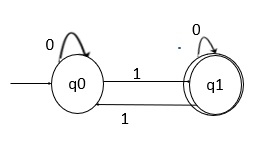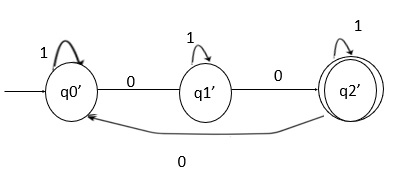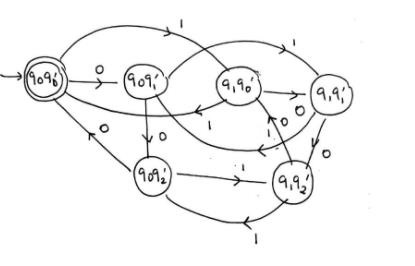# Construct a DFA recognizing the language {x | the number of 1's is divisible by 2, and 0'sby 3} over an alphabet ∑={0,1}

Data Structure AlgorithmsComputer ScienceComputers

## Problem

The given language L={ x | the number of 1's is divisible by 2, and 0's by 3} over an alphabet ∑={0,1}.

## Solution

The language is divided into two parts, first we need to find the number of 1’s divisible by 2 and second find out the number of 0’s divisible by 3, finally combine the two parts to generate a result.

Step 1 − DFA for the first part, number of 1’s divisible by 2.Here,

• q0 on 0 goes to q0 which is a final state, and generates a string 0, accepted by the given language.

• q0 on 1 goes to q1, q1 on 1 goes to q0 reaches the final state and generates a string “11” which is divisible by 2.

• q0 on 0 goes to q0, q0 on 1 goes to q1, q1 on 0 goes to q1 and q1 on 1goes to q0 which is a final state and generates a string “0101”, which is divisible by 2.

Step 2 − DFA for the second part, number of 0’s divisible by 3.Here,

• qo’ on 1 goes to q0 which is a final state and generates a string 1, accepted by the language.

• q0’ on 0 goes to q1’, q1’ on o goes to q2’ and q2’ on 0 goes to q0’ which is a final state and generates a string “000” which is divisible by 3.

Step 3 − The final DFA is: DFA first part X DFA second part.

States01
{q0q0’}{q0q1’}{q1q0’}
{q0q1’}{q0q2’}{q1q1’}
{q0q2’}{q0q0’}{q1q2’}
{q1q0’}{q1q1’}{q0q0’}
{q1q1’}{q1q2’}{q0q1’}
{q1q2’}{q1q0’}{q0q2’}

## Transition diagram

The transition diagram for the DFA is as follows −